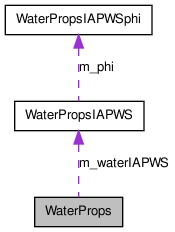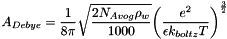# WaterProps Class Reference [Electric Properties of Phases]

The WaterProps class is used to house several approximation routines for properties of water. More...

#include <WaterProps.h>

Collaboration diagram for WaterProps:[legend]

List of all members.

## Public Member Functions

WaterProps ()
Default constructor.
WaterProps (WaterPropsIAPWS *wptr)
Constructor with pointer to Water PDSS object.
WaterProps (PDSS_Water *wptr)
Constructor with pointer to Water PDSS object.
WaterProps (const WaterProps &b)
Copy Constructor.
virtual ~WaterProps ()
destructor
WaterPropsoperator= (const WaterProps &b)
Assignment operator.
doublereal relEpsilon (doublereal T, doublereal P_pascal, int ifunc=0)
Bradley-Pitzer equation for the dielectric constant of water as a function of temperature and pressure.
doublereal ADebye (doublereal T, doublereal P, int ifunc)
ADebye calculates the value of A_Debye as a function of temperature and pressure according to relations that take into account the temperature and pressure dependence of the water density and dieletric constant.
doublereal satPressure (doublereal T)
Returns the saturation pressure given the temperature.
doublereal density_IAPWS (doublereal T, doublereal P)
Returns the density of water.
doublereal density_IAPWS () const
Returns the density of water.
doublereal coeffThermalExp_IAPWS (doublereal T, doublereal P)
returns the coefficient of thermal expansion
doublereal isothermalCompressibility_IAPWS (doublereal T, doublereal P)
Returns the isothermal compressibility of water.
doublereal viscosityWater () const
Returns the viscosity of water at the current conditions (kg/m/s).
doublereal thermalConductivityWater () const
Returns the thermal conductivity of water at the current conditions (W/m/K).

## Static Public Member Functions

static doublereal density_T (doublereal T, doublereal P, int ifunc)
Simple calculation of water density at atmospheric pressure.

## Protected Attributes

WaterPropsIAPWSm_waterIAPWS
Pointer to the WaterPropsIAPWS object.
bool m_own_sub
true if we own the WaterPropsIAPWS object

## Detailed Description

The WaterProps class is used to house several approximation routines for properties of water.

The class is also a wrapper around the WaterPropsIAPWS class which provides the calculations for the equation of state properties for water.

In particular, this class house routine for the calculation of the dielectric constant of water

Most if not all of the member functions are static.

Definition at line 111 of file WaterProps.h.

## Constructor & Destructor Documentation

 WaterProps ( )

Default constructor.

Definition at line 32 of file WaterProps.cpp.

References WaterProps::m_own_sub, and WaterProps::m_waterIAPWS.

 WaterProps ( WaterPropsIAPWS * wptr )

Constructor with pointer to Water PDSS object.

Parameters:
 wptr Pointer to WaterPropsIAPWS object

Definition at line 57 of file WaterProps.cpp.

References WaterProps::m_own_sub, and WaterProps::m_waterIAPWS.

 WaterProps ( PDSS_Water * wptr )

Constructor with pointer to Water PDSS object.

Parameters:
 wptr Pointer to water standard state object

Definition at line 44 of file WaterProps.cpp.

References PDSS_Water::getWater(), WaterProps::m_own_sub, and WaterProps::m_waterIAPWS.

 WaterProps ( const WaterProps & b )

Copy Constructor.

Copy constructor.

Parameters:
 b Object to be copied

Definition at line 73 of file WaterProps.cpp.

 ~WaterProps ( )  [virtual]

destructor

Destructor.

Definition at line 83 of file WaterProps.cpp.

References WaterProps::m_own_sub, and WaterProps::m_waterIAPWS.

## Member Function Documentation

 doublereal ADebye ( doublereal T, doublereal P, int ifunc )

ADebye calculates the value of A_Debye as a function of temperature and pressure according to relations that take into account the temperature and pressure dependence of the water density and dieletric constant.

The A_Debye expression appears on the top of the ln actCoeff term in the general Debye-Huckel expression It depends on temperature and pressure. And, therefore, most be recalculated whenever T or P changes. The units returned by this expression are sqrt(kg/gmol).Nominal value at 25C and 1atm = 1.172576 sqrt(kg/gmol).

Based on: epsilon/epsilon_0 = 78.54 (water at 25C) epsilon_0 = 8.854187817E-12 C2 N-1 m-2 e = 1.60217653E-19 C F = 9.6485309E7 C kmol-1 R = 8.314472E3 kg m2 s-2 kmol-1 K-1 T = 298.15 K B_Debye = 3.28640E9 sqrt(kg/gmol)/m Na = 6.0221415E26

Parameters:
 T Temperature (kelvin) P pressure (pascal) ifunc Changes what's returned from the routine: ifunc = 0 return value ifunc = 1 return temperature derivative ifunc = 2 return temperature second derivative ifunc = 3 return pressure first derivative
Returns:
Returns a single doublereal whose meaning depends on ifunc:
• ifunc = 0 return value
• ifunc = 1 return temperature derivative
• ifunc = 2 return temperature second derivative
• ifunc = 3 return pressure first derivative
Verification:

With the epsRelWater value from the Bradley-Pitzer relation, and the water density from the density_IAPWS() function, The A_Debye computed with this function agrees with the Pitzer table p. 99 to 4 significant digits at 25C. and 20C. (Aphi = ADebye/3)

Definition at line 321 of file WaterProps.cpp.

 doublereal coeffThermalExp_IAPWS ( doublereal T, doublereal P )

returns the coefficient of thermal expansion

Parameters:
 T Temperature (kelvin) P pressure (pascal)

Definition at line 473 of file WaterProps.cpp.

 doublereal density_IAPWS ( ) const

Returns the density of water.

This function uses the internal state of the underlying water object

Definition at line 468 of file WaterProps.cpp.

References WaterPropsIAPWS::density(), and WaterProps::m_waterIAPWS.

 doublereal density_IAPWS ( doublereal T, doublereal P )

Returns the density of water.

This function sets the internal temperature and pressure of the underlying object at the same time.

Parameters:
 T Temperature (kelvin) P pressure (pascal)

Definition at line 458 of file WaterProps.cpp.

References WaterPropsIAPWS::density(), and WaterProps::m_waterIAPWS.

 doublereal density_T ( doublereal T, doublereal P, int ifunc )  [static]

Simple calculation of water density at atmospheric pressure.

Valid up to boiling point.

static function. This formulation has no dependence on the pressure and shouldn't be used where accuracy is needed.

Parameters:
 T temperature in kelvin P Pressure in pascal ifunc changes what's returned
Returns:
value returned depends on ifunc value: ifunc = 0 Returns the density in kg/m^3 ifunc = 1 returns the derivative of the density wrt T. ifunc = 2 returns the 2nd derivative of the density wrt T ifunc = 3 returns the derivative of the density wrt P.

Verification: Agrees with the CRC values (6-10) for up to 4 sig digits.

units = returns density in kg m-3.

Definition at line 133 of file WaterProps.cpp.

References Cantera::GasConstant.

 doublereal isothermalCompressibility_IAPWS ( doublereal T, doublereal P )

Returns the isothermal compressibility of water.

Parameters:
 T temperature in kelvin P pressure in pascal

Definition at line 483 of file WaterProps.cpp.

 WaterProps & operator= ( const WaterProps & b )

Assignment operator.

Parameters:
 b Object to be copied

Definition at line 92 of file WaterProps.cpp.

References WaterProps::m_own_sub, and WaterProps::m_waterIAPWS.

 doublereal relEpsilon ( doublereal T, doublereal P_pascal, int ifunc = 0 )

Bradley-Pitzer equation for the dielectric constant of water as a function of temperature and pressure.

Returns the dimensionless relative dielectric constant and its derivatives.

Range of validity: 0 to 350C, 0 to 1 kbar pressure

Parameters:
 T temperature (kelvin) P_pascal pressure in pascal ifunc changes what's returned from the function ifunc = 0 return value ifunc = 1 return temperature derivative ifunc = 2 return temperature second derivative ifunc = 3 return pressure first derivative
Returns:
Depends on the value of ifunc:
• ifunc = 0 return value
• ifunc = 1 return temperature derivative
• ifunc = 2 return temperature second derivative
• ifunc = 3 return pressure first derivative
Validation: Numerical experiments indicate that this function agrees with the Archer and Wang data in the CRC p. 6-10 to all 4 significant digits shown (0 to 100C).

value at 25C and 1 atm, relEps = 78.38

Returns the dimensionless relative dielectric constant and its derivatives.

ifunc = 0 value ifunc = 1 Temperature deriviative ifunc = 2 second temperature derivative ifunc = 3 return pressure first derivative

Range of validity 0 to 350C, 0 to 1 kbar pressure

Parameters:
 T temperature (kelvin) P_pascal pressure in pascal ifunc changes what's returned from the function
Returns:
Depends on the value of ifunc: ifunc = 0 return value ifunc = 1 return temperature derivative ifunc = 2 return second temperature derivative ifunc = 3 return pressure first derivative

Validation: Numerical experiments indicate that this function agrees with the Archer and Wang data in the CRC p. 6-10 to all 4 significant digits shown (0 to 100C).

value at 25C, relEps = 78.38

Definition at line 218 of file WaterProps.cpp.

 doublereal satPressure ( doublereal T )

Returns the saturation pressure given the temperature.

Parameters:
 T temperature (kelvin)
Returns:
returns the saturation pressure (pascal)

Definition at line 445 of file WaterProps.cpp.

References WaterProps::m_waterIAPWS, and WaterPropsIAPWS::psat().

 doublereal thermalConductivityWater ( ) const

Returns the thermal conductivity of water at the current conditions (W/m/K).

This function calculates the value of the thermal conductivity of water at the current T and P.

The formulas used are from the paper J. V. Sengers, J. T. R. Watson, "Improved International Formulations for the Viscosity and Thermal Conductivity of Water Substance", J. Phys. Chem. Ref. Data, 15, 1291 (1986).

The formulation is accurate for all temperatures and pressures, for steam and for water, even near the critical point. Pressures above 500 MPa and temperature above 900 C are suspect.

Definition at line 615 of file WaterProps.cpp.

 doublereal viscosityWater ( ) const

Returns the viscosity of water at the current conditions (kg/m/s).

This function calculates the value of the viscosity of pure water at the current T and P.

The formulas used are from the paper J. V. Sengers, J. T. R. Watson, "Improved International Formulations for the Viscosity and Thermal Conductivity of Water Substance", J. Phys. Chem. Ref. Data, 15, 1291 (1986).

The formulation is accurate for all temperatures and pressures, for steam and for water, even near the critical point. Pressures above 500 MPa and temperature above 900 C are suspect.

Definition at line 536 of file WaterProps.cpp.

## Member Data Documentation

 bool m_own_sub [protected]

true if we own the WaterPropsIAPWS object

Definition at line 352 of file WaterProps.h.

Referenced by WaterProps::operator=(), WaterProps::WaterProps(), and WaterProps::~WaterProps().

 WaterPropsIAPWS* m_waterIAPWS [protected]

Pointer to the WaterPropsIAPWS object.

this pointer points to the water object.

Definition at line 349 of file WaterProps.h.

The documentation for this class was generated from the following files:
Generated by1.6.3